• +91 9971497814
• info@interviewmaterial.com

# Triangles EX-6.6 Interview Questions Answers

### Related Subjects

Question 1 :

In Figure, PS is thebisector of QPR of ∆ PQR. Provethat QS/PQ = SR/PR

Answer 1 :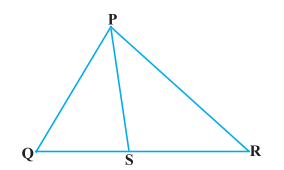Solution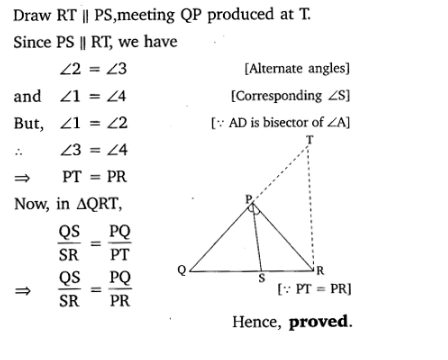Question 2 : In Fig. 6.57, D is a point on hypotenuse AC of∆ABC, such that BD AC,DM BC and DN AB.

Prove that: (i) DM_2 = DN . MC (ii) DN_2 = DM . AN.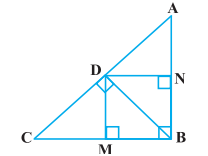Solution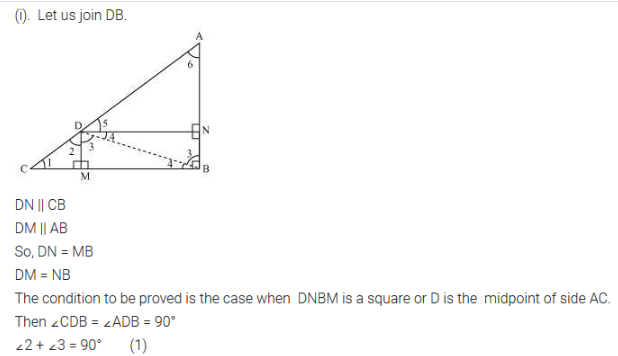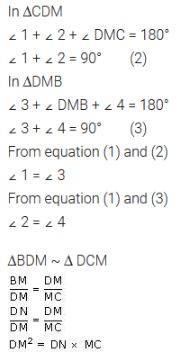Solution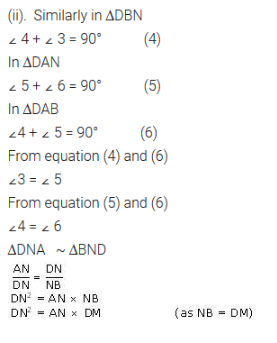Question 3 :

In Figure, ABC is atriangle in which ABC > 90° and AD CB produced. Prove that

AC2= AB2+BC2+ 2 BC.BD.

Answer 3 :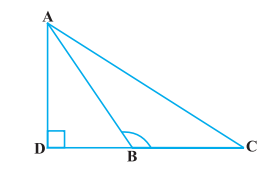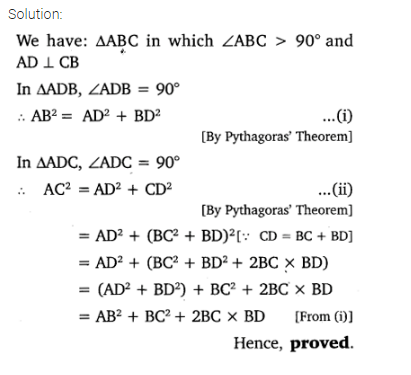Question 4 :

In Figure, ABC is atriangle in which ABC < 90° and AD BC.

Prove that

AC_2= AB_2+ BC_2 – 2 BC.BD.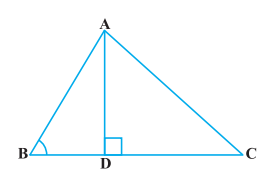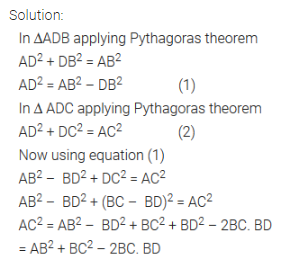Question 5 :

In Figure, AD is amedian of a triangle ABC and AM BC.

Prove that :

(i) AC_2 = AD_2 + BC.DM + 2 (BC/2)_ 2

(ii) AB_2 = AD_2 – BC.DM + 2 (BC/2)_ 2

(iii) AC_2 + AB_2 = 2 AD_2 + ½ BC_2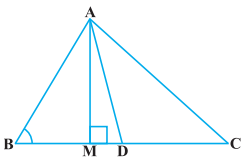Solution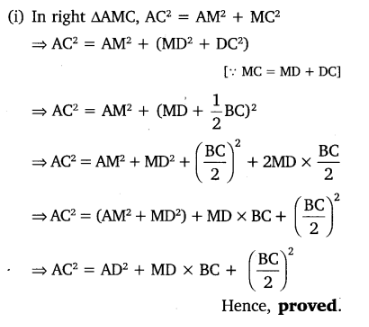Solution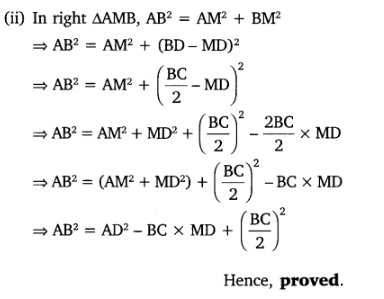Solution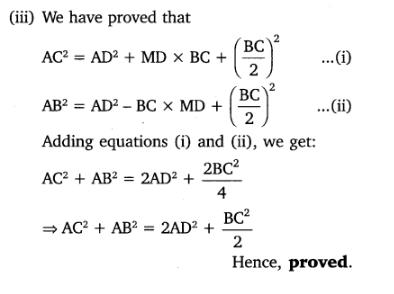Question 6 :

Prove that the sumof the squares of the diagonals of parallelogram is equal to the sum of thesquares of its sides.